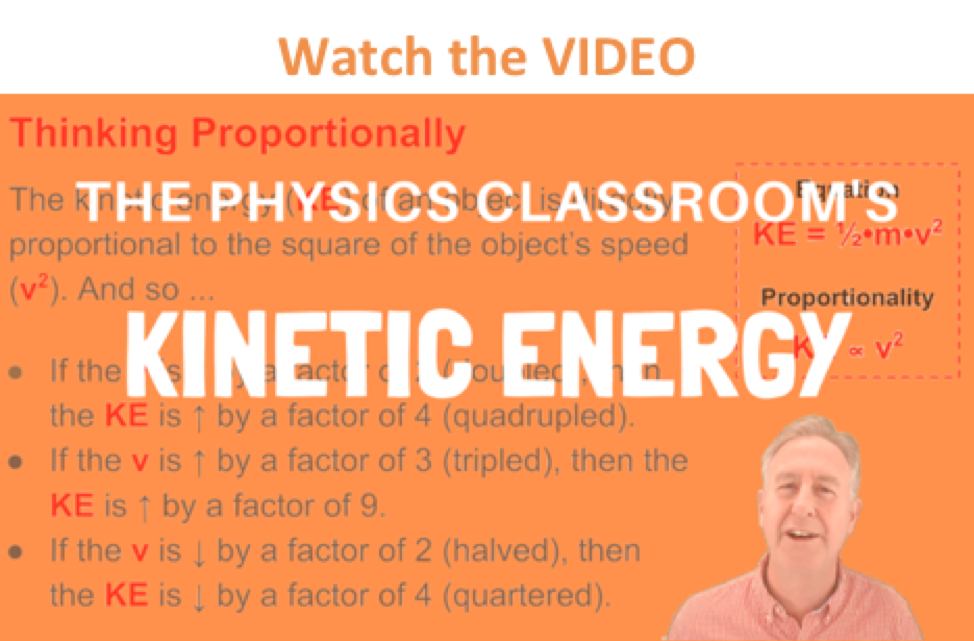Work, Energy, and Power - Lesson 1 - Basic Terminology and Concepts

# Kinetic EnergyKinetic energy is the energy of motion. An object that has motion - whether it is vertical or horizontal motion - has kinetic energy. There are many forms of kinetic energy - vibrational (the energy due to vibrational motion), rotational (the energy due to rotational motion), and translational (the energy due to motion from one location to another). To keep matters simple, we will focus upon translational kinetic energy. The amount of translational kinetic energy (from here on, the phrase kinetic energy will refer to translational kinetic energy) that an object has depends upon two variables: the mass (m) of the object and the speed (v) of the object. The following equation is used to represent the kinetic energy (KE) of an object.

KE = 0.5 • m • v2

where m = mass of object

v = speed of objectThis equation reveals that the kinetic energy of an object is directly proportional to the square of its speed. That means that for a twofold increase in speed, the kinetic energy will increase by a factor of four. For a threefold increase in speed, the kinetic energy will increase by a factor of nine. And for a fourfold increase in speed, the kinetic energy will increase by a factor of sixteen. The kinetic energy is dependent upon the square of the speed. As it is often said, an equation is not merely a recipe for algebraic problem solving, but also a guide to thinking about the relationship between quantities.

Kinetic energy is a scalar quantity; it does not have a direction. Unlike velocity, acceleration, force, and momentum, the kinetic energy of an object is completely described by magnitude alone. Like work and potential energy, the standard metric unit of measurement for kinetic energy is the Joule. As might be implied by the above equation, 1 Joule is equivalent to 1 kg*(m/s)^2.

1 Joule = 1 kg • m2/s2

### We Would Like to Suggest ...How does a car's speed (and thus its kinetic energy) affect the distance that would be required for it to brake to a stop? Interact, Explore, and Learn the answer to this question with our Stopping Distance Interactive. You can find it in the Physics Interactives section of our website. The Stopping Distance Interactive allows a learner to explore the effect of speed upon the stopping distance of a toy car.

Use your understanding of kinetic energy to answer the following questions. Then click the button to view the answers.

1. Determine the kinetic energy of a 625-kg roller coaster car that is moving with a speed of 18.3 m/s.

2. If the roller coaster car in the above problem were moving with twice the speed, then what would be its new kinetic energy?

3. Missy Diwater, the former platform diver for the Ringling Brother's Circus, had a kinetic energy of 12 000 J just prior to hitting the bucket of water. If Missy's mass is 40 kg, then what is her speed?

4. A 900-kg compact car moving at 60 mi/hr has approximately 320 000 Joules of kinetic energy. Estimate its new kinetic energy if it is moving at 30 mi/hr. (HINT: use the kinetic energy equation as a "guide to thinking.")

Next Section: